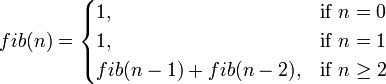# 函数式编程中的 Pattern Matching (模式匹配)

Posted on:``````fib 0 = 1
fib 1 = 1
fib n | n >= 2
= fib (n-1) + fib (n-2)``````

``````fib n | n == 0 = 1
| n == 1 = 1
| n >= 2 = fib (n-1) + fib (n-2)``````

## 和 switch/ifelse 语句的区别

1. 编译器可以替你检查你是否覆盖了所有情形
2. 可以直接把 pattern match 作为一个赋值语句
3. 如果你有一个不同类型复合的变量，每一个匹配结果都会有不同的类型
4. 使用 pattern matching 在某些情况下要简洁得多